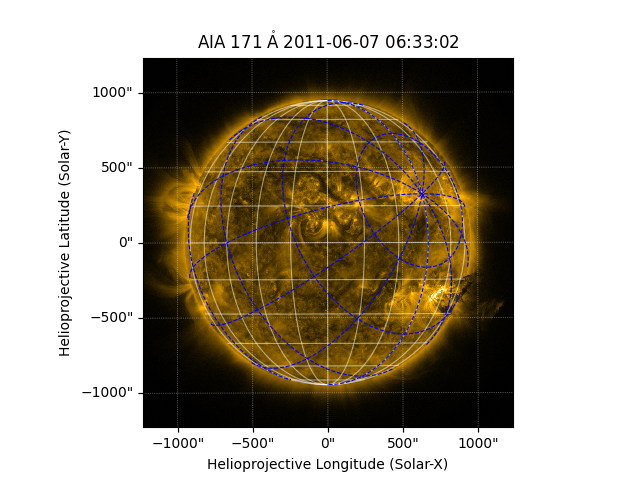# Offsetting the north pole of a coordinate frame#

How to use NorthOffsetFrame to offset the north pole for a coordinate frame.

import matplotlib.pyplot as plt

import astropy.units as u
from astropy.coordinates import SkyCoord

import sunpy.map
from sunpy.coordinates import NorthOffsetFrame
from sunpy.data.sample import AIA_171_IMAGE


For an AIA observation from the sample data, let’s create a modified Heliographic Stonyhurst coordinate frame with its north pole offset to a longitude of 45 degrees and a latitude of 20 degrees.

aiamap = sunpy.map.Map(AIA_171_IMAGE)

north = SkyCoord(45*u.deg, 20*u.deg,
frame="heliographic_stonyhurst", obstime=aiamap.date)
new_frame = NorthOffsetFrame(north=north)


Let’s plot the normal Heliographic Stonyhurst coordinate frame as a white grid and the modified coordinate frame as a blue grid. If the north pole of this new frame were placed at a point of interest, the lines of longitude form great circles radially away from the point, and the lines of latitude measure angular distance from the point.

fig = plt.figure()
aiamap.plot(axes=ax, clip_interval=(1, 99.99)*u.percent)
aiamap.draw_grid(axes=ax)

overlay = ax.get_coords_overlay(new_frame)
overlay.set_ticks(spacing=30. * u.deg)
overlay.grid(ls='--', color='blue')

plt.show()Total running time of the script: (0 minutes 0.948 seconds)

Gallery generated by Sphinx-Gallery